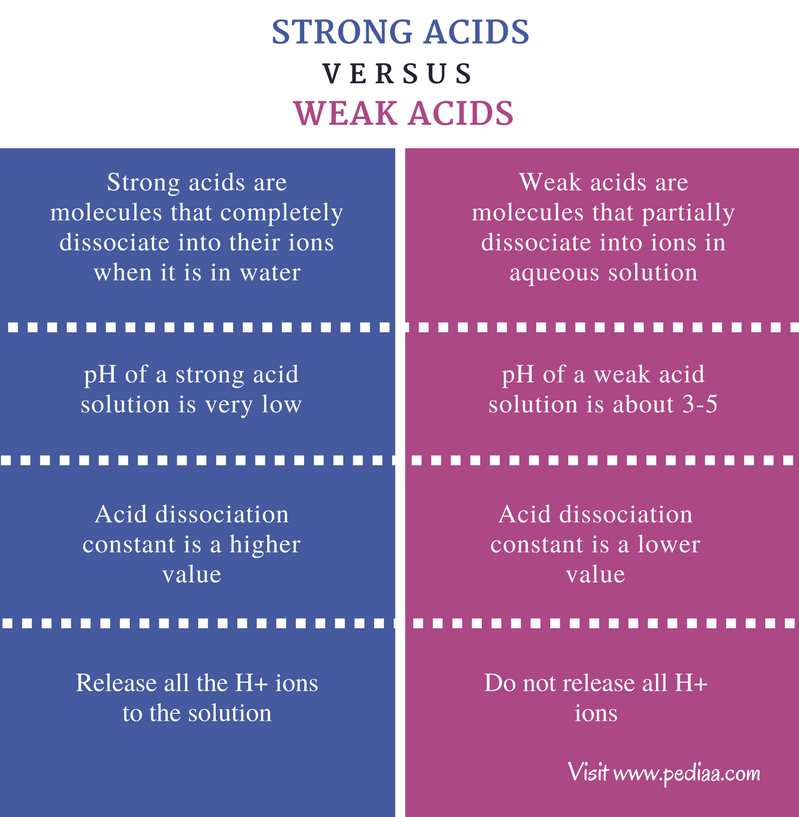# Difference Between Strong and Weak Acids

## Main Difference – Strong vs Weak Acids

An acid is a molecule or other species which can donate a proton or accept an electron pair in reactions. Acids are classified into two groups known as strong acids and weak acids. The main difference between strong and weak acids is that strong acids dissociate completely in aqueous solutions whereas weak acids partially dissociate in aqueous solutions.

### Key Areas Covered

1. What is a Strong Acid
– Definition, Properties, Examples
2. What is a Weak Acid
– Definition, Properties, Examples
3. What is the difference between Strong Acid and Weak Acid
– Comparison of Key Differences

Key Terms: Acid, Acid Dissociation Constant, Deprotonation, pH, Polarity, Proton, Strong Acid, Weak Acid## What is a Strong Acid

Strong acids are molecules that completely dissociate into their ions when it is in water. In other words, acids release H+ ions into the solution by their complete ionization. The strength of an acid is characterized by their acid dissociation constant values (Ka). Normally, strong acids have a very large Ka value.

The stronger the acid, more easily it loses protons. These protons are easily released due to the high polarity of the bond between H atom and the rest of the molecule. This polarity is determined by the electronegativity of two atoms involved in this bond. The deprotonation (removal of a proton) of a strong acid depends on the polarity and the size of the anion which the proton is attached to.

As an example, if an acid named H-A is considered, the dissociation of HA acid can be given as,

HA(aq)        +       H2O(l)         à    A(aq)          +       H3O+(aq)

But if the acid molecule has more than one protons that can be released, it can be shown as below. The below example shows the dissociation of a diprotic acid. This means it can release two protons.

H2B(aq)       +      H2O(l)        à    B2-(aq)              +      H3O+(aq)

On the other hand, the pH of the solution is greatly influenced by strong acids because strong acids release H+ ions to the solution. The pH depends on the H+ concentration. The relationship between H+ concentration and the pH can be given as below.

pH    =      -log[H+(aq)]

If the acid is a strong acid, the pH value is a very small value. For example, if a strong monoprotic acid is in water at a 0.1 molL-1 concentration, the pH of the solution would be,

pH       =       -log[H+(aq)]

pH              =      -log[0.1 molL-1  ]

=       1

### Examples of Strong Acids

• Hydrochloric acid (HCl)
• Nitric acid (HNO3)
• Sulfuric acid (H2SO4)
• Hydrobromic acid (HBr)
• Hydroiodic acid (HI)
• Perchloric acid (HClO4)
• Chloric acid (HClO3)

## What is a Weak Acid

Weak acids are molecules that partially dissociate into ions in aqueous solutions. Weak acids do not release all the H+ ions to the solution. The acid dissociation constant (Ka) is a small value than that of strong acids.  The pH of the solution is about 3-5. That is because the weak acid does not raise the H+ concentration of a solution as a strong acid does. In a system of weak acid in water, there are H+ ions, anion of the molecule and the weak acid molecule present in the solution.

For example, the dissociation of ethanoic acid can be shown as below.Figure 1: Dissociation of Ethanoic Acid

When writing the dissociation of weak acids, one should use double arrows instead of a single arrow. This is to show the reaction is an equilibrium reaction.

### Examples of Weak Acids

• Sulfurous acid (H2SO3)
• Phosphoric acid (H3PO4)
• Hydrofluoric acid (HF)
• Nitrous acid (HNO2)
• Benzoic acid (C6H5COOH)
• Formic acid (HCOOH)
• Acetic acid (CH3COOH)

## Difference Between Strong and Weak Acids

### Definition

Strong Acid: Strong acids are molecules that completely dissociate into their ions when it is in water.

Weak Acid: Weak acids are molecules that partially dissociate into ions in aqueous solution.

### pH

Strong Acid: The pH of a strong acid solution is very low (about pH=1).

Weak Acid: The pH of a weak acid solution is about 3-5.

### Acid Dissociation Constant

Strong Acid: The acid dissociation constant Ka is a higher value for strong acids.

Weak Acid: The acid dissociation constant Ka is a lower value for strong acids.

### Amount of H+ Ions Released

Strong Acid: Strong acids release all the H+ ions it can release to the solution.

Weak Acid: Weak acids do not release all the H+ ions.

### Conclusion

The strength of an acid is determined by the polarity and the atomic sizes of the acid molecule. According to the way that acid molecules dissociate in water, there are two types of acids as strong acids and weak acids. The main difference between strong and weak acids is that strong acids dissociate completely in aqueous solutions whereas weak acids partially dissociate in aqueous solutions.

##### References:

1.”Strong and weak acids .” Strong and weak acids. N.p., n.d. Web. Available here. 26 June 2017.
2.Helmenstine, Anne Marie. “What Determines the Strength of an Acid or Base?” ThoughtCo. N.p., n.d. Web. Available here. 26 June 2017.
3.”Acids and Alkalis.” GCSE CHEMISTRY – What is the Difference between Strong and Weak Acids? – GCSE SCIENCE. N.p., n.d. Web. Available here. 26 June 2017.

##### Image Courtesy:

1. “Acetic-acid-dissociation-2D” By Ben Mills – Own work (Public Domain) via Commons Wikimedia